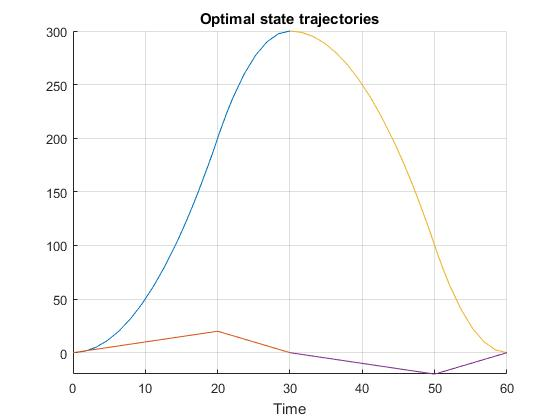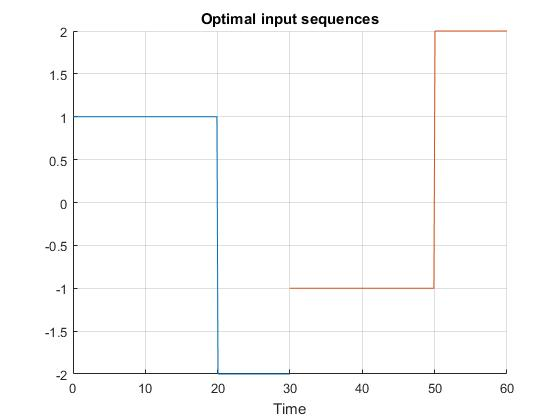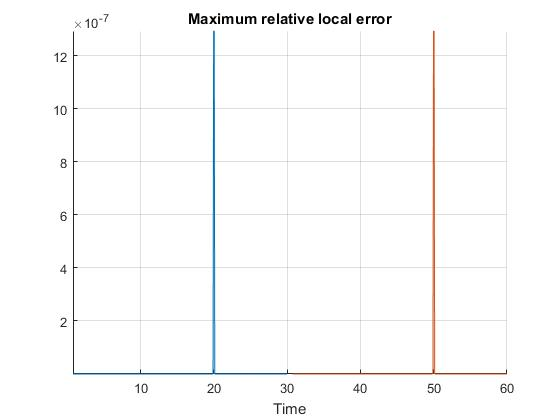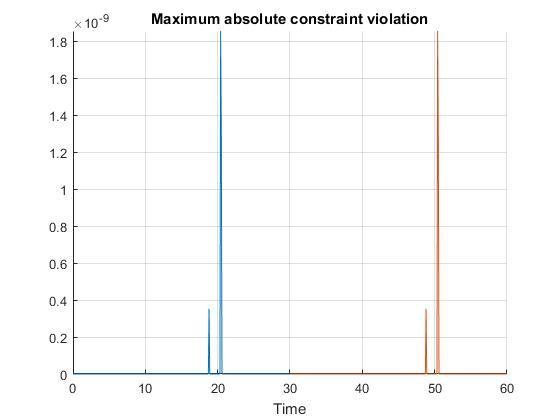Example: Bang-bang Control (Multi-Phase)

Difficulty: Intermediate

This problem is an extention to the single phase bang-bang control problem. Now, we would like to have the double integrator system to be repositioned from x=0 [m] to x=300 [m] and then back to x=0 [m] in mimimum time.

Formulate the problem in ICLOCS2

Problem definition for multiphase problem

In problem defination file BangBang.m, we can define the time variables for different phases

problem.mp.time.t_min=[0 0 0];
problem.mp.time.t_max=[0 70 70];
guess.mp.time=[0 30 60];

the static parameters (as part of optimization variables)

problem.mp.parameters.pl=[];
problem.mp.parameters.pu=[];
guess.mp.parameters=[];

and the lower bound, uppder bound and tolerance for some linear and nonlinear linkage constraints.

problem.mp.constraints.bll.linear=[0 0];
problem.mp.constraints.blu.linear=[0 0];
problem.mp.constraints.blTol.linear=[0.01 0.01];

problem.mp.constraints.bll.nonlinear=[];
problem.mp.constraints.blu.nonlinear=[];
problem.mp.constraints.blTol.nonlinear=[];

Next, we need to specify different phases of the OCP as well as the corresponding settings for each phase

[problem.phases{1},guess.phases{1}] = BangBangTwoPhase_Phase1(problem.mp, guess.mp);
[problem.phases{2},guess.phases{2}] = BangBangTwoPhase_Phase2(problem.mp, guess.mp);
phaseoptions{1}=problem.phases{1}.settings(20);
phaseoptions{2}=problem.phases{2}.settings(20);

In the linkage constraint function bclink, we can define the linkage constraint equations as

blc_linear=[xf{1}(1)-x0{2}(1);xf{1}(2)-x0{2}(2)];
blc_nonlinear=[];

The option file for the multiphase problem is settings_BangbangTwoPhase.m. The structure of the file is the same as settings_Bangbang.m for the single-phase bang-bang control problem, but only contains global settings.

Problem definition for individual phases

First, the problem definition for the individual phases can be specified following the same guideline as for single-phase problems. For this particular example, we generated the following files

• BangBang_Dynamics_Phase1.m: same as BangBang_Dynamics_Internal.m
• BangBangTwoPhase_Phase1.m: same as BangBang.m, with minor differences. The main ones are for the time variables
% Initial time.
problem.time.t0_idx=1;
problem.time.t0_min=problem_mp.time.t_min(problem.time.t0_idx);
problem.time.t0_max=problem_mp.time.t_max(problem.time.t0_idx);
guess.t0=guess_mp.time(problem.time.t0_idx);

% Final time. Let tf_min=tf_max if tf is fixed.
problem.time.tf_idx=2;
problem.time.tf_min=problem_mp.time.t_min(problem.time.tf_idx);
problem.time.tf_max=problem_mp.time.t_max(problem.time.tf_idx);
guess.tf=guess_mp.time(problem.time.tf_idx);
for static parameters,
% Parameters bounds.
problem.parameters.pl=problem_mp.parameters.pl;
problem.parameters.pu=problem_mp.parameters.pu;
guess.parameters=guess_mp.parameters;
for the data storage static parameters
problem.data=problem_mp.data;
and in the Mayer cost function E_unscaled
boundaryCost=0;
• BangBangTwoPhase_Phase2.m: same as BangBang.m, with minor differences. The main ones are for the time variables
% Initial time.
problem.time.t0_idx=2;
problem.time.t0_min=problem_mp.time.t_min(problem.time.t0_idx);
problem.time.t0_max=problem_mp.time.t_max(problem.time.t0_idx);
guess.t0=guess_mp.time(problem.time.t0_idx);

% Final time. Let tf_min=tf_max if tf is fixed.
problem.time.tf_idx=3;
problem.time.tf_min=problem_mp.time.t_min(problem.time.tf_idx);
problem.time.tf_max=problem_mp.time.t_max(problem.time.tf_idx);
guess.tf=guess_mp.time(problem.time.tf_idx);
for static parameters,
% Parameters bounds.
problem.parameters.pl=problem_mp.parameters.pl;
problem.parameters.pu=problem_mp.parameters.pu;
guess.parameters=guess_mp.parameters;
for state variables,
% Initial conditions for system.
problem.states.x0l=[0 -200];
problem.states.x0u=[300 200];

% State bounds
problem.states.xl=[0 -200];
problem.states.xu=[310 200];

% Terminal state bounds.
problem.states.xfl=[0 0];
problem.states.xfu=[0 0];

% Guess the state trajectories with [x0 xf]
guess.states(:,1)=[100 0];
guess.states(:,2)=[-50 0];
for input variable,
% Input bounds
problem.inputs.ul=[-1];
problem.inputs.uu=;

% Bounds on first control action
problem.inputs.u0l=[-1];
problem.inputs.u0u=;

% Guess the input sequences with [u0 uf]
guess.inputs(:,1)=[-1 2];
and for the data storage static parameters
problem.data=problem_mp.data;
• hessianLagrangian_BangBang.m: same as hessianLagrangian_BangBang.m
• jacConst_BangBang.m: same as jacConst_BangBang.m
• settings_BangbangTwoPhase_Phase1.m: same as settings_Bangbang.m, but only contains phase depended settings
• settings_BangbangTwoPhase_Phase2.m: same as settings_Bangbang.m, but only contains phase depended settings

Main script main_BangbangTwoPhase.m for solving the multi-phase bang-bang control problem

Now we can fetch the problem and options, solve the resultant NLP, and generate some plots for the solution.

[problem,guess,options.phaseoptions]=BangBangTwoPhase;          % Fetch the problem definition
options.mp= settings_BangbangTwoPhase;                  % Get options and solver settings
[solution,MRHistory]=solveMyProblem( problem,guess,options);
genSolutionPlots(options, solution);

Results from ICLOCS2

Using the Hermite-Simpson discretization scheme of ICLOCS2, the following state and input trajectories are obtained under a mesh refinement scheme starting with 20 mesh points for each phase. The computation time with IPOPT (NLP convergence tol set to 1e-09) is about 1 seconds with one mesh refinement iteration altogether, using analytical derivative calculations on an Intel i7-6700 desktop computer. The graphs are generated by ICLOCS 2.5 built-in genSolutionPlots command.Figure 1: Optimal state trajectory for the multiphase bang-bang control problemFigure 2: Optimal input trajectory for the multiphase bang-bang control problemFigure 3: Co-state trajectory for the multiphase bang-bang control problemFigure 4: Maximum absolute local error for the multiphase bang-bang control problemFigure 5: Maximum relative local error for the multiphase bang-bang control problemFigure 6: Maximum constraint violation error for the multiphase bang-bang control problem